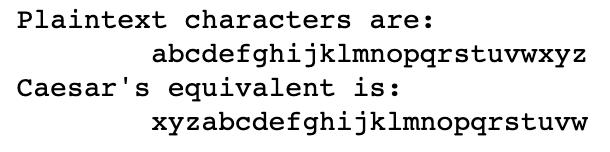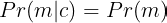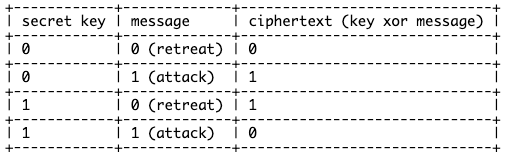# Shift cipher

In the shift cipher (a.k.a Caesar’s cipher), two parties A and B agree on a common key, a number in between 0 to 24, this key is secret and they don’t share it with anybody else. In the shift cipher the key is translated into the number of jumps on the alphabet, let’s take a key (shift) of 3 as an example. The substitution of letters would be:Caesar’s cipher substitution for key k=3

# Better security by increasing the key space

We’ve seen that if the key space (number of possible secret keys) is small it is not difficult to find the correct key and then decrypt all the messages. So, we can increase this key size and then the probability for the attacker to find the correct key is reduced (or, he’ll have to invest a lot of time to find it).

# Leaking information from ciphertexts

In the mono-alphabetic cipher it may be difficult for the attacker to get the exact key but still he can get some valuable information by just looking at the ciphertexts. Imagine that the attacker knows the language of communication in between the two parties (Alice and Bob) and so by just observing the ciphertexts he can infer information from the messages. Imagine this language is plain english, the attacker then knows that some words are more frequent than others, for instance “the”, “be”, “to”, “of” or “and” are listed as the most frequent words in english. This means that the attacker will observe many times the words “dco”, “vo”, “dk”, “ki” and “agb” in the ciphertexts (if we use the substitution cipher introduced in the example). Now he can exploit that to find some letter substitutions in the key.

# Perfect secrecy and the one time pad

Can we find a way such that the ciphertext does not contain any information?. First let me write a definition (a more formal definition can be found in the book of Katz and Lindell) . An encryption scheme is considered to be perfectly secret ifAn encryption scheme is perfectly secret if the probability of a message does not change by the observation of a ciphertext.XOR operation for the one time pad for messages of 1 bit.
• The key has to be at least as long as the message one wants to transmit. This means we have to store a lot of information. For instance if we transmit a text message in ASCII encoding (8 bits per letter) and send a word of 10 letters we would need a key of 80 bits at least.
• For perfect secrecy one has to use a new key every time. When Alice and Bob meet in person they will have to exchange a lot of keys and use one by one sequentially for their communications. This again is a lot of information and is impractical.
• Alice and Bob have to make sure that they are the only ones that know the key. In the examples I always stated that they have to meet in person, this is a way to make sure that nobody is spying them. When using computers one wants to establish a secure communication through an insecure channel like the internet between two computers that are physically very far away. This makes the one time pad totally impractical.

# Improving the one time pad: stream ciphers

The general idea of the one time pad is used in stream ciphers. Here we need the notion of a pseudorandom generator (see Introduction to modern cryptography from Katz and Lindell), this is an algorithm that inputs a number (a.k.a the seed) and outputs what looks like a random string of bits. In essence a good pseudorandom generator (PRG) must output bit strings that are difficult to distinguish from pure random.

# Improving the stream cipher using quantum physics

Now imagine that Alice and Bob could generate the same pure random streams of bits for a moment. For an attacker eavesdropping all the communications between Alice and Bob the ciphertexts would look totally random, that’s the case of perfect secrecy!. Don’t overreact but we are close to find the perfect cipher!. Now the question. Can we generate pure correlated randomness between Alice and Bob?

# Takeaways from this post

We presented the shift cipher and the substitution cipher as simple examples to illustrate the problem of the ciphertext carrying information from the message. We’ve seen with a simple attack on those ciphers how can one get information from the messages by just observing the ciphertexts. Then stated the problem of getting ciphertexts not containing any information from the message, i.e. perfect secrecy and we’ve seen that even though we can achieve it with the one time pad this is not a good practical solution for several reasons. A more practical approach is to use stream ciphers where take the same philosophy of padding the message with a random stream of bits. Here however we use pseudo-random generators to generate the noise, and the problem is that this noise is not purely random so stream ciphers are not perfectly secure but practical in many applications such as mobile communications. One way to make stream ciphers perfectly secure is to generate the randomness using quantum physics, an active field of research nowadays.

--

--